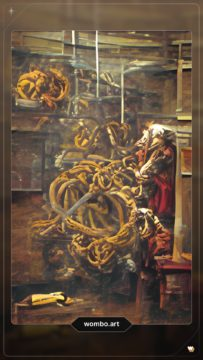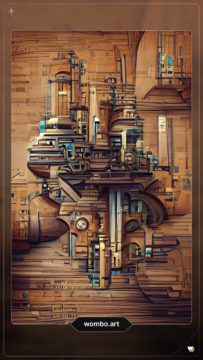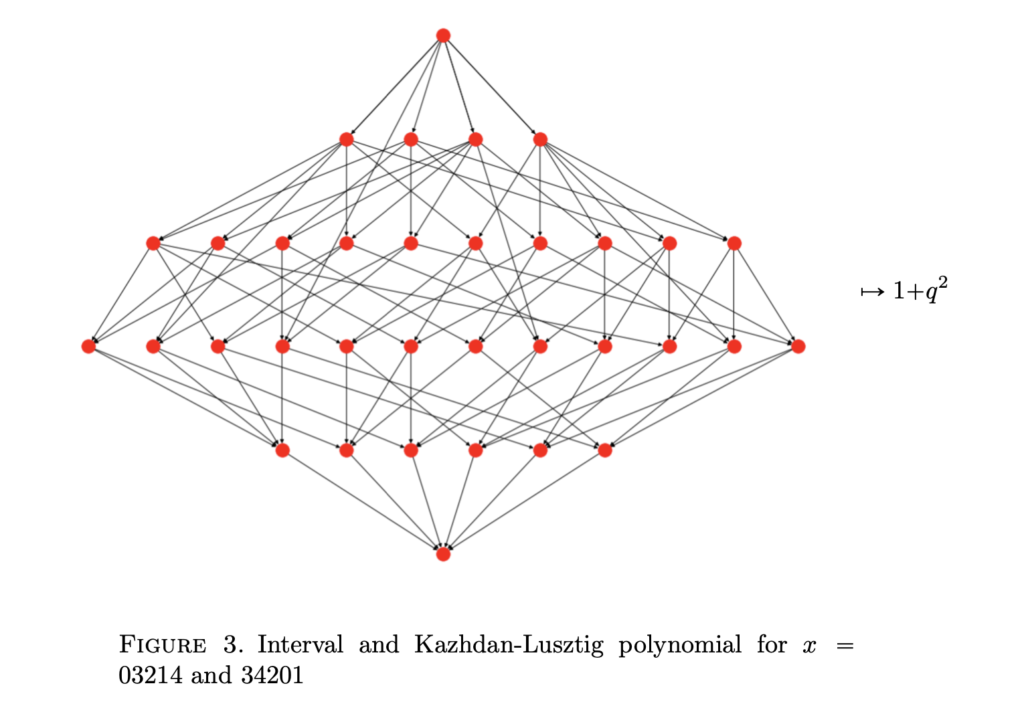# Do androids dream of mathematics?

by Jonathan KujawaImage from In a research project with Brian Boe and Dan Nakano at the University of Georgia some years ago we computed the following list of numbers :

2, 3, 6.

There is one of these numbers for each pair of numbers m and n. The 2 is from when m and n both equal 1, the 3 was when m equals 2 and n equals 1, and the 6 was from when m and n both equal 2. The 6 took several days and was about the limit of what we could compute by hand.

By analogy from similar calculations in other situations, it was our belief that there should be a reasonable formula that could compute these numbers just given m and n. But that was faith and intuition, not science — and definitely not math! Despite the Law of Small Numbers, mathematicians are firm believers that coincidences rarely happen. Given data, there should be an understandable order and pattern. Certainly 2000+ years of mathematics support this optimism.

In my work with Brian and Dan, we ended up proving that the above list of numbers is given by the formula (where r is the smaller of m and n):

(m+n)*r – r² + r.

If you would like to see the details, see formula (1.1.2) in this paper. We never had more than those three numbers for data, but through a combination of theoretical considerations and plain ol’ guessing, we were able to figure out the pattern.

In trying to solve other research questions you sometimes have the opposite problem. You can generate lots of data, even huge amounts of data, but it is hard to see the forest for the trees.

In the old days, this data had to be generated by hand. People like Fermat, Gauss, and Euler were absolute machines when it came to lengthy, tedious calculations. In 1742 Goldbach wrote a letter to Euler and conjectured that every number could be written as the sum of three primes. He had no doubt calculated many, many examples, and Euler no doubt computed many, many more. But no matter how many examples you calculate, the natural numbers are inexhaustible.

If you don’t have a proof, you can at least generate more data. In 1938, Nils Pipping checked Goldbach’s conjecture for every number between 1 and 100,000! Fortunately, nowadays we have computers. According to Wikipedia, Goldbach’s conjecture has been verified up to 4,000,000,000,000,000,000. Still no proof, though .

For nearly 2000+ years, if you wanted guidance on how to interpret data you looked to the work of other mathematicians. Mathematics is built brick-by-brick on others’ results. By looking at what others have done you hopefully gain insight into what could be true in your situation and get ideas for what strategies might solve the problem. If Brian, Dan, and I didn’t have prior work to inspire us, we would have had little confidence that only m and n should be needed in our formula. After all, there could always be hidden variables or even no pattern at all. Fortunately, we weren’t completely in the dark.  We had light to work by thanks to those who came before us.

Now we have powerful computers. Great news! More data! For example, some of my colleagues contribute to a modern version of Pipping’s hand calculations, the L-functions and modular forms database.

Unfortunately, big data can mean big problems. You can generate a vast amount of data, but that might only make the problem all the more impenetrable. Fortunately, math and computers have also given us new tools for discovering patterns in large data sets. In 2015 we talked here at 3QD about how you can use the tools of topology to find the hidden structure of large data collections. Topology is the field of math that studies geometry, but focuses on connections and rough shapes rather than fine details. It’s perfect for determining the shape of noisy, high-dimensional data.

Another way to find patterns in data is to use machine learning. This is the AI that is used to recognize cat pictures, translate from one language to another, detect lung cancer in MRI scans, drive cars down the freeway, and loads more. If you want to distinguish between different pictures, or predict what sentence in French will convey the same meaning as “Cat on a hot tin roof,” you give the AI a bunch of training data of what you would like it to be able to do (*these* are pictures of cats, and *these* are pictures of not-cats), and it will find its way to rules on how to get from point A to point B.

A truly remarkable example of what AI can do is OpenAI’s Codex software. It turns plain English into sophisticated computer code. See here, here, and here for examples of what it can do. It just came out and will only improve. I showed it to my Calculus students as a warning against career plans based on being a mindless cubical drone for 20-30 years.  Computers keep doing things previously thought impossible.

In a pair of essays last summer, I wrote about how computer tools were starting to revolutionize mathematical research. In those essays, it was about the role of proof verification software. A kind of spell- and grammar-checker for mathematics. I speculated that it wasn’t too much farther to have a virtual research assistant. A digital dæmon that could sit on your shoulder, point out possible errors and give potentially helpful suggestions. It would already be great to have a mathematical Codex who could be asked in plain English to write a proof by induction of some complicated formula.

But none of this is the creative side of mathematics. The intuition, the noticing of possible patterns, the ability to make insightful conjectures. Can computers help here as well?Image from .In December 2021 there was an article in the journal Nature where theoretical mathematicians teamed up with researchers at DeepMind to see if AI could be used to generate new and interesting insights into difficult mathematical problems. They used AI plus lots of human creativity to make significant new progress on two problems . Since both problems touch the mathematics of my own research, I was immediately interested.

The first problem is in the area of knot theory. We’ve talked about the mathematics of knots here at 3QD. Probably only sailors and mathematicians appreciate how complicated knots can be. In mathematics, there are several radically different approaches to the study of knots. One method involves the study of hyperbolic geometry related to the knot, while a second approach involves tools from physics and algebra (that’s where my research comes in). The important point is that these two methods are completely different. There are no obvious connections between the two. Given a knot, each of them gives you various “invariants” of the knot. The invariants in each theory are different and describe different features of the knot.

Nevertheless, when the two theories are used to study the same knot you might hope that there could be some relationship between the invariants they generate. This relationship was what Davies, Juhasz, Lackenby, and Tomasev hoped the computer could extract from big data sets.

By giving the AI large data sets of randomly chosen knots the computer predicted that a new hyperbolic quantity called the natural slope should have a close relation to an invariant from the physics/algebra theory called the “signature”. Not only were the mathematicians able to use the AI to predict the previously unsuspected relationship, they were able to prove it! Motivated by the data they also came up with other interesting results and conjectures about the role of the natural slope of a knot.

The second problem was in representation theory. Kazhdan-Lusztig polynomials are important polynomials that play a fundamental role in both algebra and geometry . They were discovered in the 1980s and have played a central role in mathematics ever since.Image from .On the one hand, these polynomials aren’t so mysterious. We have algorithms for computing them and have databases of, literally, millions of Kazhdan-Lustig polynomials. On the other hand, these algorithms are recursive: to compute one Kazhdan-Lusztig polynomial requires that you first compute all the earlier ones. The New York Times thought it was worthy to write an article about the massive effort it took to compute all the Kazhdan-Lusztig polynomials in one special case.

It would be nice to have more direct ways of computing the polynomials and, more importantly, a better understanding of their structure and the role they play. This was the goal of the work of Blundell, Buesing, Davies, Velickovic, and Williamson. They hoped AI could help shed light on a long-standing conjecture about Kazhdan-Lusztig polynomials.

Let me briefly describe what they did. For each natural number n, the symmetric group Sn is the collection of all rearrangements of n distinct objects. We saw the symmetric group here at 3QD when we talked about the mathematics of rearranging DNA. One thing we didn’t talk about at the time is the Bruhat graph of the symmetric group. This is a geometric object which describes how different elements of the symmetric group are related. Here is an image of part of the Bruhat graph in an example:Part of a Bruhat graph from the paper by [BBDVW].In this picture, each red dot is one of the symmetries in the symmetric group. The black lines connect closely related symmetries, and the graph is drawn so that the simpler symmetries are towards the bottom of the picture. The Bruhat graph is a skeleton of the original symmetric group. A huge amount of information is lost by only looking at the Bruhat graph.

For each pair of symmetries a and b in the symmetric group, there is a Kazhdan-Lusztig polynomial pa,b(q). The Combinatorial Invariance Conjecture predicts that the Kazhdan-Lusztig polynomial depends only on the geometry of the Bruhat graph and, more precisely, only depends on the part of the graph which is between the dot for a and the dot for b. In the picture above, the Kazhdan-Lusztig polynomial is q² + 1 and we should be able to compute that just from looking at this picture. As it stands, our algorithms compute pa,b(q) using much more information.

This conjecture was made independently by Lusztig and Dyer in the 1980s and has resisted all attempts at proof (or disproof!). Millions of examples have been computed, the conjecture checks out in every case, but until now it was mostly a mystery.

The researchers pointed the AI at all this data and asked it to predict what features of the Bruhat graph are key to the computation of Kazhdan-Lusztig polynomials. The computer pointed them to a new way of decomposing the Bruhat graph into smaller pieces, which the researchers call a “hypercube decomposition”. They were able to prove that a hypercube decomposition does indeed give you enough information to compute the Kazhdan-Lusztig polynomial (remember, the computer only makes predictions, not promises!).

This doesn’t quite prove the Combinatorial Invariance Conjecture. These hypercube decompositions do seem to be the key, and the researchers have a precise conjecture now for how to use them to prove the Combinatorial Invariance Conjecture. If nothing else, they now know where to look! In this video, Geordie Williamson gives an overview of his perspective on their project.

Like the Hubble and Webb telescopes, computers give us powerful new tools for gathering and interpreting data, and for verifying results once we think we’ve figured them out. It’s an exciting new era in math research.

That said, mathematics is a deeply human and creative activity. I have no worry that I’ll be Codexed out of a job anytime soon!

 All images are generated by this AI. Top to bottom, I used the phrases “three quarks daily”, “knots, machines, and computation”, and “symmetry, geometry, and shape”.

 In my area of math, there is a number that measures the complexity of a mathematical object. This measure is imaginatively called complexity. In our calculations, 2, 3, and 6 were the complexities of gl(1|1), gl(2|1), and gl(2|2).

 There is a weaker form of Goldbach’s conjecture which is that every odd number can be written as the sum of three primes. This was proven by Harold Helfgott ~6 years ago. I’m not an expert, but my understanding is that his proof is generally believed, but it has not yet appeared in a journal in final form. See his webpage for the latest.

 The Nature paper is mostly a meta-description of the collaboration. If you’d like to see the gory details of the math itself, the knot theory paper can be found here and the Kazhdan-Lusztig polynomial paper can be found here.

 Remember, a polynomial is a function which has a rule something like: p(q) = q6 + 3 q5 + 12 q4 + q2 + q + 7. For historical reasons, it is customary to use the variable q when talking about Kazhdan-Lusztig polynomials.